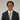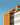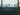Navigate back to the homepage

Mocking with d6tflowJack Moody
October 25th, 2020 · 3 min readIntro to d6tflow

Recently I was introduced to the concept of data science pipelines. In data science workflows (for machine learning, especially), there are many different steps where data is transformed, cleaned, and prepared for training. Stringing a sequence of functions can lead to very messy code. The d6tflow package takes an OOP approach to this problem that intelligently handles dependent tasks.

If you are working with large datasets, it may also take some time to run all of the code to clean your data from scratch. It would be nice to have a way to save intermediate data and load it.

The d6tflow package offers the ability to take care of this for you. If you are familiar with the luigi project by Spotify and are wondering how you might apply a similar workflow to data science, give d6tflow a try. Go check out the d6tflow getting started guide or take a look at the documentation to get familiar with the package. In this article, I will assume some basic knowledge of d6tflow. If you want to learn more about d6tflow before learning how to make mock objects for unit testing, check out my article introducing d6tflow.

Predicting Apple Stock Price Movement

To show a very simple application of d6tflow, I will be looking to see if we can predict Apple's (NYSE: AAPL) stock returns tomorrow based on its returns today. We are not interested in the actual price of the stock as much as the actual percentage return. We will try to predict tomorrow's percentage return based on today's percentage return and see if there is any pattern.

To keep things simple, I will use the sklearn package and use a linear regression model. I have sourced price data for Apple from Yahoo Finance. The data used in this article can be found at this GitHub repository.

The first task of any data science pipeline is obtaining and cleaning data. I already downloaded Apple pricing data in a CSV file from Yahoo Finance to simplify things.

1import d6tflow
2import luigi
3import pandas as pd
4from sklearn.linear_model import LinearRegression
5from sklearn.model_selection import train_test_split
6
7
9 filename = luigi.Parameter(default='../data/AAPL.csv')
10
11 def run(self):
13 aapl_df.columns = aapl_df.columns.str.replace(' ', '_').str.lower()
14 self.save(aapl_df)

Each task needs to have a run method. This method tells d6tflow what it needs to do during the task. Here, we read the CSV file with the pricing data, rename the columns by replacing all spaces with underscores and make everything lowercase. Finally, we save the data frame we just created and transformed to a parquet file.

Now let's preprocess the data to get ready for training.

3 persist = ['X', 'y', 'aapl_df']
4
5 def run(self):
7 # Calculate daily adj close pct change
9
10 # Want to predict tomorrow's return % based on today's return %
14 self.save({'X': X, 'y': y, 'aapl_df': aapl_df})

A few things are going on here from d6tflow. Firstly, d6tflow is requiring that the LoadData task has been run. Once d6tflow knows that LoadData has ran, it loads the output of the task. We can now pick up where we left off in the last task. Because we want to predict what tomorrow's return will be, we calculate the percentage change between the adjusted closing prices. Out of curiosity, let's see if today's volume has any effect on today's return.

We line up yesterday's returns and today's volume in X with tomorrow's returns in y.

Now comes the fun part! We fit a basic linear regression model using yesterday's returns and today's volume to predict today's returns.

1@d6tflow.requires(PreprocessData)
3 persist = ['X_test', 'y_test', 'reg']
4
5 def run(self):
8
9 reg = LinearRegression()
10 X_train, X_test, y_train, y_test = train_test_split(
11 X, y, test_size=0.2, random_state=0)
12 reg.fit(X_train, y_train)
13 self.save({
14 'X_test': X_test,
15 'y_test': y_test,
16 'reg': reg
17 })

Again, we need to use @d6tflow.requires to require that the data has been preprocessed. We load the features (X) and the response (y) and fit the regression on part of the data, keeping some aside for out-of-sample testing.

Now that our model is trained, let's see how we did. How much information does yesterday's returns give about today's expected returns.

1@d6tflow.requires(FitData)
3 def run(self):
4 # See how well the regression fits the test data
8
9 score = reg.score(X_test, y_test)
10 self.save(score)

Up to this point, we haven't actually ran any of our pipeline. However, this is very easy to do using d6tflow. All we need to do is tell d6tflow to run the ScoreFit task. It will find and run all of the dependencies for us. Let's keep the script to run the pipeline in the same file and only run the pipeline if we explicitly tell Python to run the file.

1if __name__ == '__main__':
2 d6tflow.run(ScoreFit())
4 print(f'Score of regression was {score:.2f%}')

After running the pipeline, we find that we get an r-squared of approximately -0.11. That is pretty close to zero.

Conclusion

It is perhaps trivial that a company's stock price would not be

What are Mocks?

A lot of times, when you are working with data science pipelines, you are going to have a lot of data. However, you don't need all of that data to test the functionality of tasks in your d6tflow pipeline.

To see how mocks work in action, let's consider a concrete example. Say we want to

More articles from Jack MoodyUsing d6tflow in Python to Simplify Data Science Pipelines and Develop Faster

Clean your data science pipelines and develop faster with d6tflow.

November 9th, 2020 · 3 min readMocking with d6tflow

Use mocks to test d6tflow pipelines.

October 25th, 2020 · 3 min read
Link to $https://stackoverflow.com/users/8206432/jack-moodyLink to$https://github.com/jackmoody11